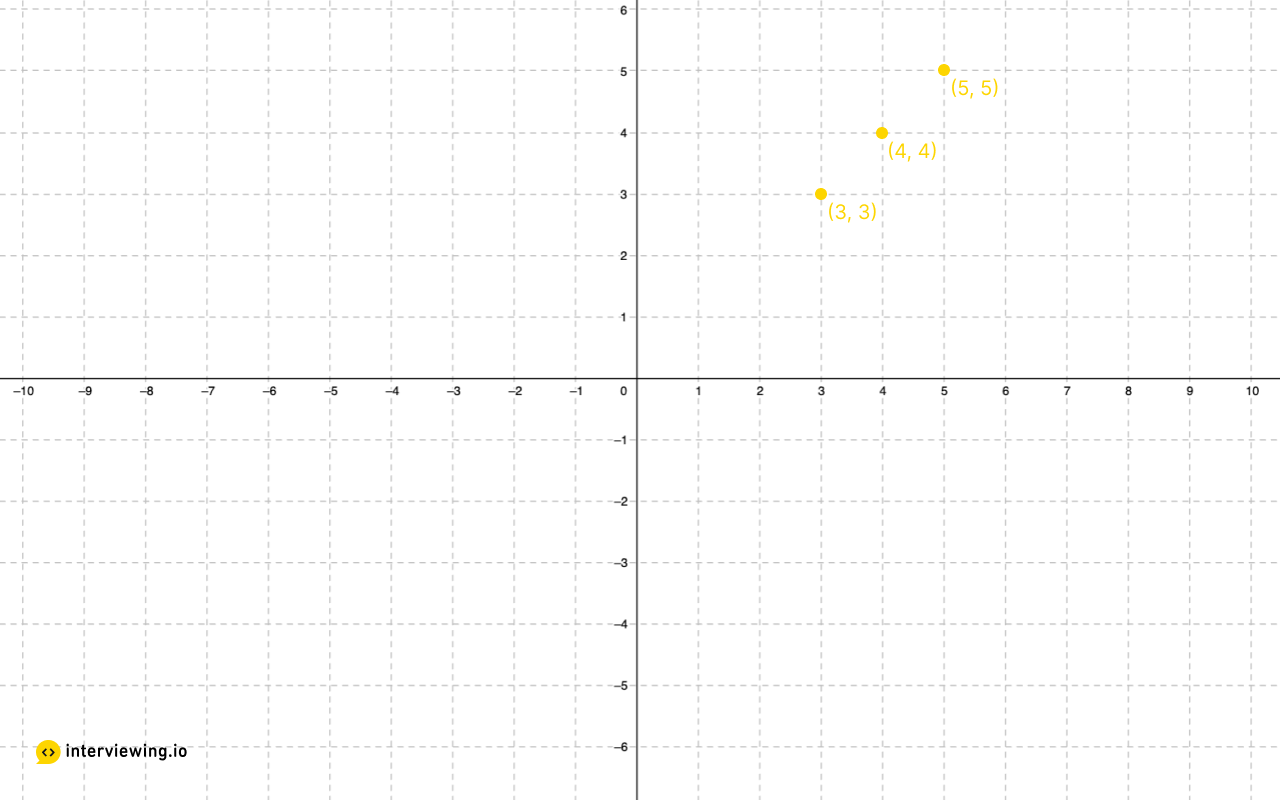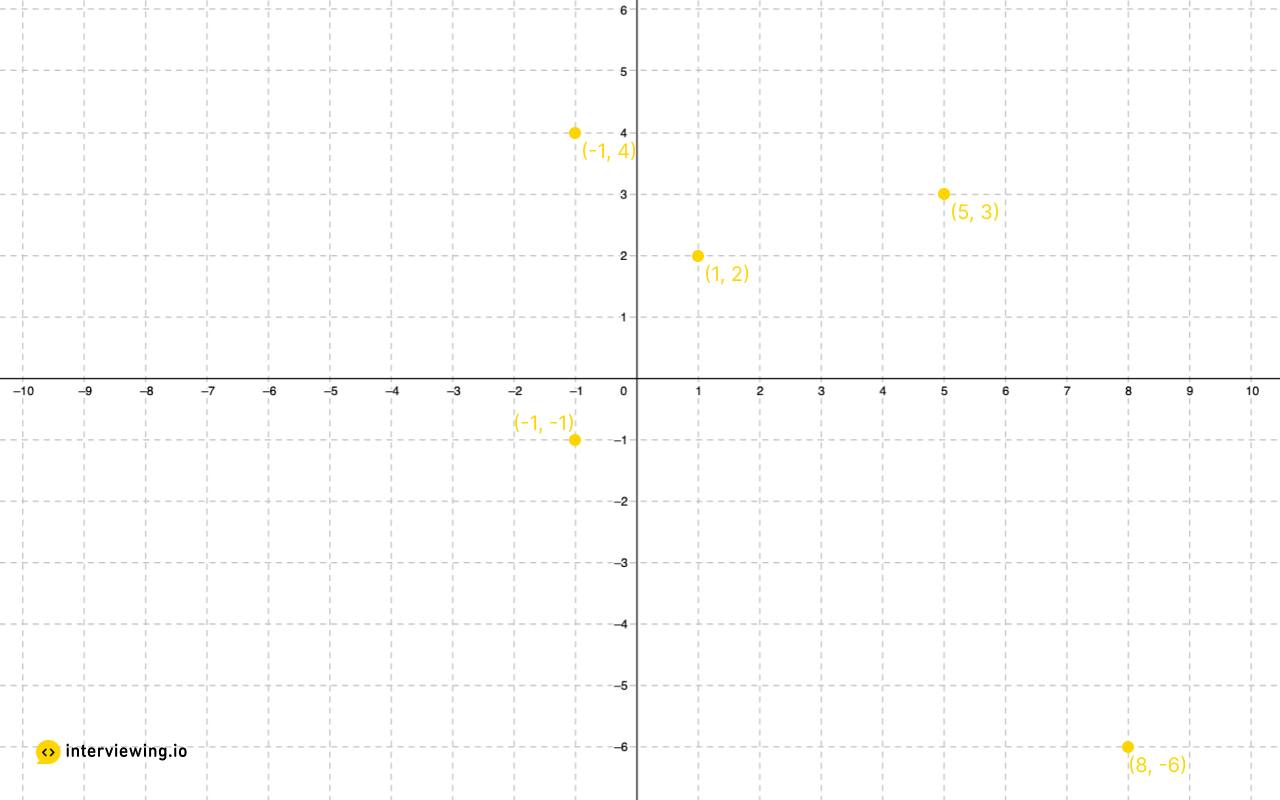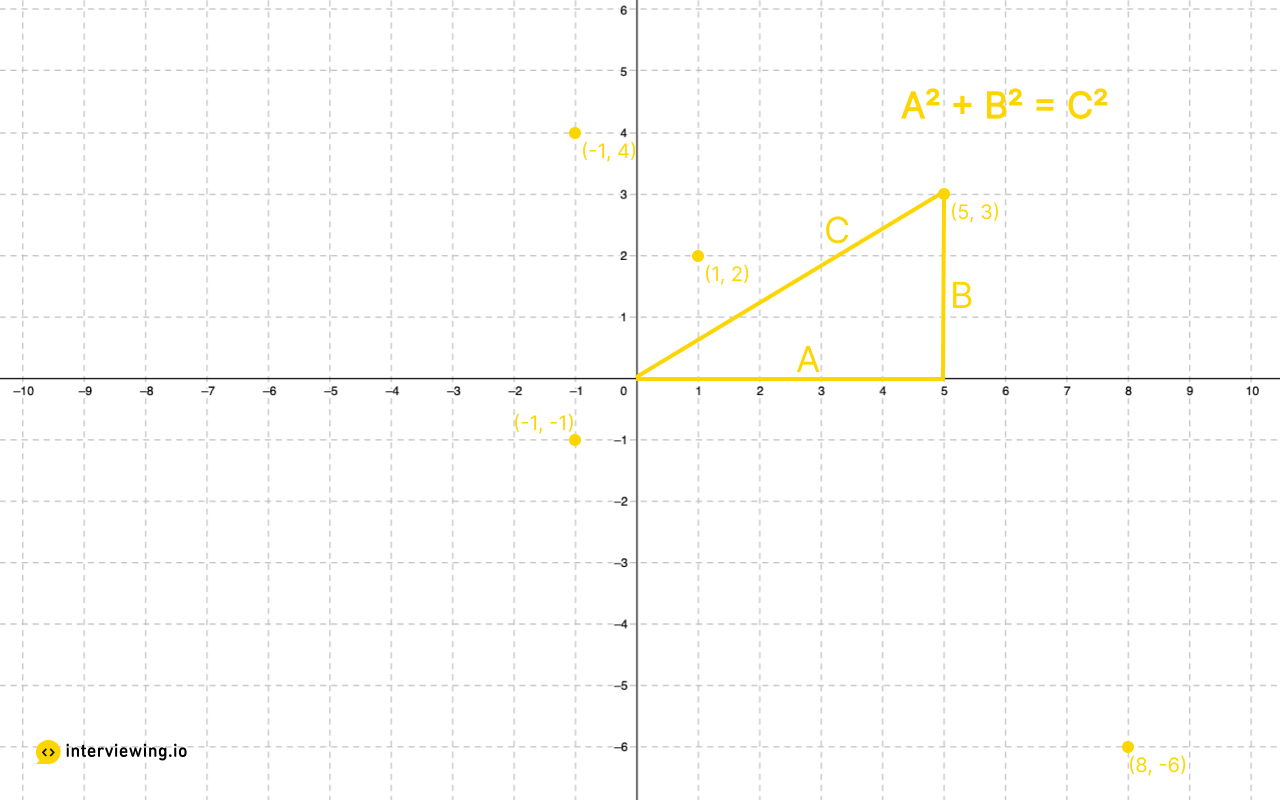MEDIUM
DATA STRUCTURES AND ALGORITHMS

# How to Solve K Closest Points To Origin

Written ByTom WagnerKenny Polyak

## K Closest Points To Origin Introduction

The K Closest Points To Origin problem involves comparing the distance of points plotted on a graph. This is a common problem in data analysis, most often found in the context of generating nearest neighbor sets. Similar to other k-selection algorithms, this problem can be solved with a variety of sorting techniques and challenges us to use a heap data structure to improve time complexity. Before viewing the problem and solution, below are some short video snippets from real mock interviews to help prepare you for some common pitfalls that interviewees stumble into.

### • Interview Analysis: Snippets from Real Interviews 🔥

1. Common Mistakes: Forgetting Data Structures
Even if you do a good job otherwise, forgetting what a certain data structure looks like could be grounds for failure. Watch this example to see what a real interviewer thinks when this happens.

2. Common Mistakes: Syntax Errors
Interviewees often know more than one programming language. Before you interview, you should familiarize yourself with the language you will be interviewing in, especially if it differs from the one you use at work.

3. Common Mistakes: Variable Names and Data Types
Variables and data types are foundational concepts, but experienced engineers still make these simple mistakes.

4. Senior Level Extension Question
See what separates a senior engineer from a junior/mid-level.

5. Possible Approach: Checking Distance
Find out how a senior engineer recommends checking distance in this question. It'll surprise you.

6. Optimization: Size Efficiency
You've heard the phrase "Size isn't everything, it's how you use it." That couldn't be more true for this question!

## K Closest Points To Origin Problem

Given a list of tuples that represent (X, Y) coordinates on an XY plane and an integer K, return a list of the K-closest points to the origin (0, 0).

### Example Inputs and Outputs

#### Example 1

Input:
points = [[5, 5], [3, 3], [4, 4]], k = 2
Output:
[[3, 3], [4, 4]] or [[4, 4], [3, 3]]#### Example 2

Input:
points = [[-1, 4], [5, 3], [-1, -1], [8, -6], [1, 2]], k = 2
Output:
[[-1, -1], [1, 2]] or [[1, 2], [-1, -1]]Constraints
The number of nodes in the list is in the range [0, 5000]
K is >= 0 and <= the length of the input list

## K Closest Points To Origin Solutions

Reading through the problem a few ideas immediately jump out:

1. We need to calculate the distance of each point from the origin (or at a minimum convert the coordinate tuple to a numerical value)
2. Once the distance has been calculated we need to determine the K-closest points

To solve this problem we will need to do some basic algebra. We have a right triangle and we need to calculate the length of the hypotenuse, and we can do so using the Pythagorean theorem:

`A^2 + B^2 = C^2`As a quick aside, we don't actually need to calculate `C` (the hypotenuse, or distance from origin), as simply calculating `A^2 + B^2` for each coordinate will allow us to order the points from closest to furthest from origin without actually determining the exact distance.

Now that we know how to calculate the distances, let's explore different ways to find the k-closest points.

#### 1. Sorting

Given we need to find the `K` closest points to origin, the naive approach should hopefully become clear relatively quickly. If we calculate the distance for each coordinate pair, we can then sort the coordinates by distance and finally slice the list from 0 to `K` in order to return the `K` closest points to the origin.

#### K Closest Points to Origin Python and JavaScript Solutions - Sorting

``````def kClosest(self, points: List[List[int]], k: int) -> List[List[int]]:
# Note: Sorting the input list mutates the input
# -> whether the input should be mutated (as opposed to copied and then sorted)
# -> can be decided collaboratively with the interviewer
points.sort(key=lambda xy_tuple: xy_tuple**2 + xy_tuple**2)
return points[:k]``````

#### Time/Space Complexity Analysis

• Time Complexity: `O(n * log(n))`
• Space Complexity: `O(k)`, as we are sorting in place and returning a new list with `K` points

#### 2. Using a Heap

Given we have found a somewhat-obvious, naive solution that runs in `O(n*log(n))` time, we should start thinking about how we can use different approaches or data structures in order to optimize the time complexity of our algorithm. In order to improve our time complexity we will need to avoid fully sorting the input, and if we aren't sorting the input we will need to repeatedly select the point with the smallest distance from the origin.

It is here that alarm bells should start to go off in our head. What data structure can be used to efficiently, repeatedly select the smallest (or largest) item in a collection? And the answer is... a heap!

To give a quick refresher, heaps are an ordered (but not fully sorted) data structure often backed by an array. They can be created in linear time and they ensure selection of the smallest or largest element at any given time, but they are not fully in order. Read more about how heaps are constructed and used here.

Back to our problem, we can iterate over the list, calculate the distance from the origin for each coordinate and convert it to a heap in place. From there, in order to find the `K` closest points to the origin we will need to `pop` from the heap `K` times, which is often a method exposed via heap library code in a given language.

#### K Closest Points to Origin Python and JavaScript Solutions - Using a Heap

``````import heapq
def kClosest(self, points: List[List[int]], k: int) -> List[List[int]]:
# O(n)
distance_coordinate_tuples = [(x*x + y*y, [x, y]) for x, y in points]
# O(n)
heapq.heapify(distance_coordinate_tuples)
# O(k*log(n))
k_smallest = heapq.nsmallest(k, distance_coordinate_tuples)
# O(k)
return [coordinate for distance, coordinate in k_smallest]
# same as above as a one-liner
def kClosest(self, points, k):
return heapq.nsmallest(k, points, key=lambda p: p**2 + p**2)``````

#### Time/Space Complexity Analysis

• Time Complexity: `O(n) + O(k*log(n))`
• Space Complexity: `O(1)` (or `O(n)` if you mutate the input)

## Practice the K Closest Points To Origin Problem With Our AI Interviewer

Start AI Interview

## K Closest Points To Origin Analysis

#### Common Mistakes: Forgetting Data Structures

Often candidates may have a great understanding of programming and problem solving skills. However if you haven't practised in a while, you may find you have forgotten what some data structures look like or how to fully implement them. In this snippet the interviewer notes that the candidate has a good understanding of algorithms and data structures but struggles with mapping those concepts onto the STL libraries. Noting the fact that the candidate had to look up what a priority queue looked like. In a real interview, this can definitely hinder your chances of passing even though you certainly have the knowledge and capabilities. Practise makes perfect, so ensure you brush up before you interview!

#### Common Mistakes: Syntax Errors

Prospective candidates will often be knowledgeable in more than one programming language. During mock technical interviews, it is important to practise and familiarize yourself with the language you will be interviewing in, especially if it differs from the native language you code in at your current job. In this snippet the the candidate notes that they more comfortable with C++ even though they mainly work with Java on the job. The interviewer points out some syntax errors in the candidate's code, specifically with creating a vector, and suggests a simpler solution. The interviewer speculates that the candidate may be trying to apply Java syntax to their C++ code.

#### Common Mistakes: Variable Names and Data Types

When calculating distance in this question, it is important to use the double data type as opposed to int. This snippet shows the importance of looking out for small mistakes in variable data types and names that can lead to errors when running code.

#### Senior Level Extension Question

Many solutions to the K closest points question do not take into account what would happen if given a large data set of points or a near infinite stream of points. A MapReduce is one solution to this problem. At a mid to senior level there is an expectation that candidates understand how to use a MapReduce. In this example after much deliberation and guidance, the candidate suggests using a MapReduce, but was unsure of the specifics and unable to implement one. The interviewer noted that the candidate's lack of experience with MapReduce could be a concern for a mid-level position, emphasizing the importance of distributed systems in handling large amounts of data. This scenario highlights the practical challenges of scaling algorithms to real-world data and the need for senior-level expertise in handling such issues.

#### Possible Approach: Checking Distance

In this snippet the interviewer suggests checking if it's the first k instead of checking if the distance is less than the minimum. This is a key tip when tackling similar problems - Often candidates rush into creating a loop and forget to add code to check if you have K.

#### Optimization: Size Efficiency

In this snippet the candidate suggests optimizing the comparator function by using a map to store distance-to-pair values, rather than recalculating distances each time. The interviewer discuss the potential challenges of working with larger data sets and to explore ways to keep the data size efficient, such as storing only the distance and index of the original point.